This tutorial is on another common toon shading technique called halftone shading, unlike normal shading it only uses full lit or full unlit as colors, but it doesn’t create a hard cut either. Instead it uses a pattern to decide which pixels are lit and which aren’t and the chance of a pixel being lit gets higher the brighter the pixel would be with a normal lighting method. To understand this tutorial I recommend reading and understanding the tutorial about custom lighting methods and the tutorial about generating screenspace texture coordinates.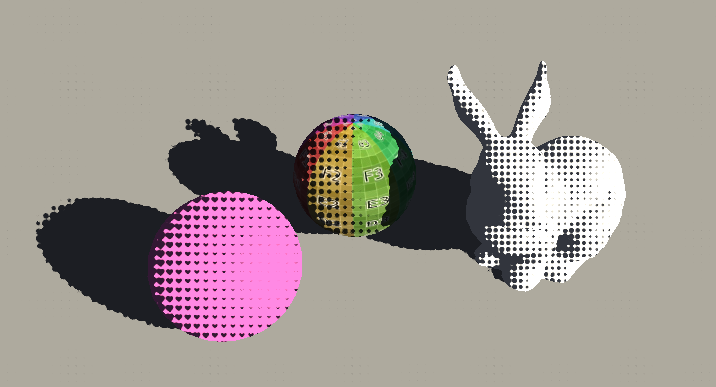For the first simplest implementation we use the result of the custom surface lighting tutorial as the base shader and start to modify that. First we make it simpler by removing the part where the shader reads the value of the toon ramp. Instead we multiply the shadow attenuation earlier with the `towardsLight` variable and pass it to the `saturate` function to clamp it between 0 and 1. Additionally the light intensity is now only saved as a one-dimensional float since we don’t read from a texture with colors anymore.

``````Shader "Tutorial/40_DitheredLighting" {
//show values to edit in inspector
Properties {
_Color ("Tint", Color) = (0, 0, 0, 1)
_MainTex ("Texture", 2D) = "white" {}
[HDR] _Emission ("Emission", color) = (0,0,0)
}
//the material is completely non-transparent and is rendered at the same time as the other opaque geometry
Tags{ "RenderType"="Opaque" "Queue"="Geometry"}

CGPROGRAM

//the shader is a surface shader, meaning that it will be extended by unity in the background to have fancy lighting and other features
//our surface shader function is called surf and we use our custom lighting model
#pragma target 3.0

sampler2D _MainTex;
fixed4 _Color;
half3 _Emission;

//our lighting function. Will be called once per light
float4 LightingCustom(SurfaceOutput s, float3 lightDir, float atten){
//how much does the normal point towards the light?
float towardsLight = dot(s.Normal, lightDir);
//remap the value from -1 to 1 to between 0 and 1
towardsLight = towardsLight * 0.5 + 0.5;

//combine shadow and light and clamp the result between 0 and 1
float lightIntensity = saturate(atten * towardsLight);

//combine the color
float4 col;
//intensity we calculated previously, diffuse color, light falloff and shadowcasting, color of the light
col.rgb = lightIntensity * s.Albedo * _LightColor0.rgb;
//in case we want to make the shader transparent in the future - irrelevant right now
col.a = s.Alpha;

return col;
}

//input struct which is automatically filled by unity
struct Input {
float2 uv_MainTex;
};

//the surface shader function which sets parameters the lighting function then uses
void surf (Input i, inout SurfaceOutput o) {
//sample and tint albedo texture
fixed4 col = tex2D(_MainTex, i.uv_MainTex);
col *= _Color;
o.Albedo = col.rgb;

o.Emission = _Emission;
}
ENDCG
}
FallBack "Standard"
}
``````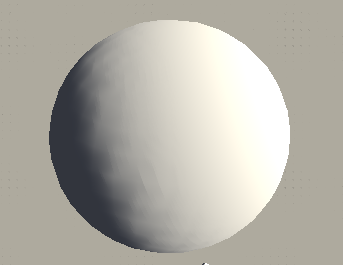With this done, we already have a value that represents how much a given pixel is lit, the next step is to change it from a gradient to a binary one or zero value. To do this we have to compare the value to another value. For this tutorial, we’re getting this other value by sampling a texture via screenspace texture coordinates. You can also use other texture coordinates like the normal UV coordinates, triplanar coordinates or even completely procedural shapes for this that don’t rely on textures at all, but I’ve found screenspace coordinates to be robust and versatile so I’m gonna use them as a example for this tutorial.

In the tutorial about screenspace coordinates I explain how to get the screenspace coordinates. As soon as we created them, we have to get them from our surface function to our lighting function. For that purpose we have to create a new surface output struct with all of the data we need. This data includes the base color of the shader, the screenspace texture coordinate, the emission of the material, the alpha transparency and the normal. Not all of those values might seem important to us right now, but the alpha and emission variables for example are needed for unity to be able to successfully generate the final shader.

``````sampler2D _HalftonePattern;
float4 _HalftonePattern_ST;
``````
``````struct HalftoneSurfaceOutput {
fixed3 Albedo;
float2 ScreenPos;
half3 Emission;
fixed Alpha;
fixed3 Normal;
};
``````
``````//input struct which is automatically filled by unity
struct Input {
float2 uv_MainTex;
float4 screenPos;
};
``````
``````//the surface shader function which sets parameters the lighting function then uses
void surf(Input i, inout HalftoneSurfaceOutput o) {
//sample and tint albedo texture
fixed4 col = tex2D(_MainTex, i.uv_MainTex);
col *= _Color;
o.Albedo = col.rgb;

o.Emission = _Emission;

float aspect = _ScreenParams.x / _ScreenParams.y;
o.ScreenPos = i.screenPos.xy / i.screenPos.w;
o.ScreenPos = TRANSFORM_TEX(o.ScreenPos, _HalftonePattern);
o.ScreenPos.x = o.ScreenPos.x * aspect;
}
``````

After transmitting those values to the lighting function we can use them to read from the new texture and write the texture values to the screen. I used a texture with several circular gradients which I generated with shadron, but you can use any tiling gradient (I also use a heart shaped signed distance field in the examples which I generated using catlikecodings signed distance field generator: https://assetstore.unity.com/packages/tools/utilities/sdf-toolkit-free-50191).

``````//our lighting function. Will be called once per light
float4 LightingHalftone(HalftoneSurfaceOutput s, float3 lightDir, float atten){

//get halftone comparison value
float halftoneValue = tex2D(_HalftonePattern, s.ScreenPos).r;

return halftoneValue;
}
``````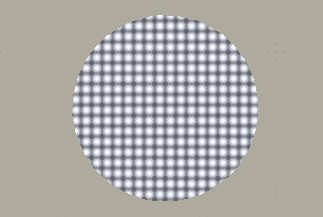Now that we have both the light intensity and the halftone comparison value we can compare them with the step function to get a binary 0 or 1 value based on the pattern of our texture. The first argument of the step function is the halftone value we just sampled from the texture and the second argument is the lightness value. If the lightness is brighter than the halftone texture value, the shader sets to pixel to be fully lit and if it’s less it’s treated as shadowed. The result of the step value is put back into the `lightIntensity` value.

``````//our lighting function. Will be called once per light
float4 LightingHalftone(HalftoneSurfaceOutput s, float3 lightDir, float atten){
//how much does the normal point towards the light?
float towardsLight = dot(s.Normal, lightDir);
//remap the value from -1 to 1 to between 0 and 1
towardsLight = towardsLight * 0.5 + 0.5;

//combine shadow and light and clamp the result between 0 and 1 to get light intensity
float lightIntensity = saturate(atten * towardsLight);

//get halftone comparison value
float halftoneValue = tex2D(_HalftonePattern, s.ScreenPos).r;

//make lightness binary between hully lit and fully shadow based on halftone pattern.
lightIntensity = step(halftoneValue, lightIntensity);

//combine the color
float4 col;
//intensity we calculated previously, diffuse color, light falloff and shadowcasting, color of the light
col.rgb = lightIntensity * s.Albedo * _LightColor0.rgb;
//in case we want to make the shader transparent in the future - irrelevant right now
col.a = s.Alpha;

return col;
}
``````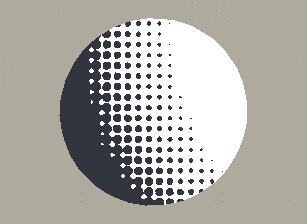## Antialiased Halftone Pattern

This works well, but we’re not actually limited by binary colors in our shaders. That means we can preserve the appearance of the halftone binary values while still using greyscale values between them to make the result look less choppy. For this we replace our `step` function with a `smoothstep` function and interpolate the colors over a single pixel. The first task is to figure out how much the value we compare to changes over a single pixel. Luckily shaders provide us with the `fwidth` function which returns a approximation of exactly that value. We divide the value of a halftone by two and then do the smoothstep from the comparison value minus half of the change where the result will be zero to the comparison value plus half of the change where the result will be one. The value we use to step between those values is the light intensity, just like previously.

``````//make lightness binary between fully lit and fully shadow based on halftone pattern (with a bit of antialiasing between)
float halftoneChange = fwidth(halftoneValue) * 0.5;
lightIntensity = smoothstep(halftoneValue - halftoneChange, halftoneValue + halftoneChange, lightIntensity);
``````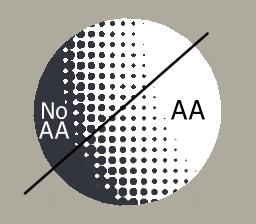## Remapping Comparison Values

If we want to change how much of the shading is shadowed and how much is illuminated we can change our texture, but this is a slow and indirect process. For quick prototyping we can also remap the values of the texture via a few uniform variables we can expose. In image manipulation programs, this process is often referred to as adjusting levels. We’re going to write a external function for this which will take the halftone comparison value as a argument as well as the input minimum value, the input maximum value, the output minimum value and the output maximum value. Apart from the first value all additional arguments are new properties we add to the shader. The minimum and maximum values don’t mean that we’re not allowed to pass values to the function that are outside of those ranges, it merely means that for input value that is the same value as the minimum input value the function will generate a output value that’s equal to the minimum output value and similarly for the maxmimum values. Values that are not those fixed values are interpolated linearly, so a input value thats exactly in the middle between the min and max input values leads to a output value thats exactly between the output minimum and maximum values.

``````_RemapInputMin ("Remap input min value", Range(0, 1)) = 0
_RemapInputMax ("Remap input max value", Range(0, 1)) = 1
_RemapOutputMin ("Remap output min value", Range(0, 1)) = 0
_RemapOutputMax ("Remap output max value", Range(0, 1)) = 1
``````
``````float _RemapInputMin;
float _RemapInputMax;
float _RemapOutputMin;
float _RemapOutputMax;
``````
``````//make lightness binary between fully lit and fully shadow based on halftone pattern (with a bit of antialiasing between)
halftoneValue = map(halftoneValue, _RemapInputMin, _RemapInputMax, _RemapOutputMin, _RemapOutputMax);
float halftoneChange = fwidth(halftoneValue) * 0.5;
lightIntensity = smoothstep(halftoneValue - halftoneChange, halftoneValue + halftoneChange, lightIntensity);
``````

Then we write the remapping function. The function consists of two parts, first we get the relative position of the input value by first subtracting the input minimum to make the value based on zero and then we divide it by the range of the input values which we can calculate by subtracting the minimum from the maximum. This relative value will be between 0 and 1 if the input value is between the minumum and maximum values, but is also able to represent values outside of that range. With this value we can then do a linear interpolation from the output minimum to the output maximum values and return the result of that.

``````float map(float input, float inMin, float inMax, float outMin,  float outMax){
float relativeValue = (input - inMin) / (inMax - inMin);
return lerp(outMin, outMax, relativeValue);
}
``````This now allows us to change which parts are counted as shadowed and which are lit in a quick and dynamic manner.

## Source

``````Shader "Tutorial/40_DitheredLighting" {
//show values to edit in inspector
Properties{
_Color("Tint", Color) = (0, 0, 0, 1)
_MainTex("Texture", 2D) = "white" {}
[HDR] _Emission("Emission", color) = (0,0,0)

_HalftonePattern("Halftone Pattern", 2D) = "white" {}

_RemapInputMin ("Remap input min value", Range(0, 1)) = 0
_RemapInputMax ("Remap input max value", Range(0, 1)) = 1
_RemapOutputMin ("Remap output min value", Range(0, 1)) = 0
_RemapOutputMax ("Remap output max value", Range(0, 1)) = 1
}
//the material is completely non-transparent and is rendered at the same time as the other opaque geometry
Tags{ "RenderType" = "Opaque" "Queue" = "Geometry"}

CGPROGRAM

//the shader is a surface shader, meaning that it will be extended by unity in the background to have fancy lighting and other features
//our surface shader function is called surf and we use our custom lighting model
#pragma target 3.0

//basic properties
sampler2D _MainTex;
fixed4 _Color;
half3 _Emission;

sampler2D _HalftonePattern;
float4 _HalftonePattern_ST;

///remapping values
float _RemapInputMin;
float _RemapInputMax;
float _RemapOutputMin;
float _RemapOutputMax;

//struct that holds information that gets transferred from surface to lighting function
struct HalftoneSurfaceOutput {
fixed3 Albedo;
float2 ScreenPos;
half3 Emission;
fixed Alpha;
fixed3 Normal;
};

// This function remaps values from a input to a output range
float map(float input, float inMin, float inMax, float outMin,  float outMax)
{
//inverse lerp with input range
float relativeValue = (input - inMin) / (inMax - inMin);
//lerp with output range
return lerp(outMin, outMax, relativeValue);
}

//our lighting function. Will be called once per light
float4 LightingHalftone(HalftoneSurfaceOutput s, float3 lightDir, float atten) {
//how much does the normal point towards the light?
float towardsLight = dot(s.Normal, lightDir);
//remap the value from -1 to 1 to between 0 and 1
towardsLight = towardsLight * 0.5 + 0.5;
//combine shadow and light and clamp the result between 0 and 1
float lightIntensity = saturate(towardsLight * atten).r;

//get halftone comparison value
float halftoneValue = tex2D(_HalftonePattern, s.ScreenPos).r;

//make lightness binary between fully lit and fully shadow based on halftone pattern (with a bit of antialiasing between)
halftoneValue = map(halftoneValue, _RemapInputMin, _RemapInputMax, _RemapOutputMin, _RemapOutputMax);
float halftoneChange = fwidth(halftoneValue) * 0.5;
lightIntensity = smoothstep(halftoneValue - halftoneChange, halftoneValue + halftoneChange, lightIntensity);

//combine the color
float4 col;
//intensity we calculated previously, diffuse color, light falloff and shadowcasting, color of the light
col.rgb = lightIntensity * s.Albedo * _LightColor0.rgb;
//in case we want to make the shader transparent in the future - irrelevant right now
col.a = s.Alpha;

return col;
}

//input struct which is automatically filled by unity
struct Input {
float2 uv_MainTex;
float4 screenPos;
};

//the surface shader function which sets parameters the lighting function then uses
void surf(Input i, inout HalftoneSurfaceOutput o) {
//set surface colors
fixed4 col = tex2D(_MainTex, i.uv_MainTex);
col *= _Color;
o.Albedo = col.rgb;

o.Emission = _Emission;

//setup screenspace UVs for lighing function
float aspect = _ScreenParams.x / _ScreenParams.y;
o.ScreenPos = i.screenPos.xy / i.screenPos.w;
o.ScreenPos = TRANSFORM_TEX(o.ScreenPos, _HalftonePattern);
o.ScreenPos.x = o.ScreenPos.x * aspect;
}
ENDCG
}
FallBack "Standard"
}
``````

As always thank you so much for reading and supporting me, your messages of support mean the world to me 💖.

You can also find me on twitter at @totallyRonja. If you liked my tutorial and want to support me you can do that on Patreon (patreon.com/RonjaTutorials) or Ko-Fi (ko-fi.com/RonjaTutorials).